﻿ 城市快速路网交通拥挤水平的非线性模糊综合评价研究 An Improved Non-Linear Fuzzy Comprehensive Method for Assessing Urban Freeway Network Traffic Congestion Level

Open Journal of Transportation Technologies
Vol. 09  No. 02 ( 2020 ), Article ID: 34649 , 11 pages
10.12677/OJTT.2020.92009

An Improved Non-Linear Fuzzy Comprehensive Method for Assessing Urban Freeway Network Traffic Congestion Level

Chunxiao Dong, Hongyun Sun*

Business School, Shandong University of Technology, Zibo Shandong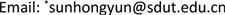Received: Feb. 26th, 2020; accepted: Mar. 13th, 2020; published: Mar. 20th, 2020ABSTRACT

The monitoring and evaluation of traffic congestion on urban freeway network is of great significance for the formulation of traffic congestion management and control strategies, one improved non-linear fuzzy comprehensive evaluation methodology (iNFCM) is proposed for urban freeway network traffic congestion assessment, which manages to highlight both the effect of judgment preference and the effect of influencing importance by incorporating new parameters. The data-driven whole assessment process consists of two sequential stages: one being calculation of network-level traffic congestion indicators; the other one being determination of network traffic congestion level. Finally, the proposed methodology, as well as traditional fuzzy comprehensive evaluation method (tFCM) and nonlinear fuzzy comprehensive method (NFCM), are applied using Shanghai urban freeway network traffic flow data collected on 2013/02/05. It is found that the exactness and stability of results obtained by iNFCM are better than those given by the other two methods.

Keywords:Urban Freeway Network, Traffic Congestion Indicator System, Non-Linear Fuzzy Comprehensive Evaluation, Judgment Preference, Influencing Importance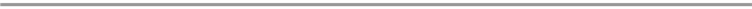1. 引言

2. 快速路网交通拥挤指标体系构建

2.1. 快速路网交通拥挤评价指标选择Figure 1. Urban freeway network traffic congestion indicator system

2.2. 各指标定义及计算

$SP=\frac{1}{K}\underset{k=1}{\overset{K}{\sum }}\underset{i\in I}{\sum }ws{p}_{i}\ast S{P}_{i}^{k}$

$S{P}_{i}^{k}=\frac{\underset{j\in {A}_{l}^{k}}{\sum }{l}_{j}}{\underset{j\in A}{\sum }{l}_{j}}$

$TP=\frac{1}{K}\underset{k=1}{\overset{K}{\sum }}\underset{i\in I}{\sum }wt{p}_{i}\ast T{P}_{i}^{k}$

$T{P}_{i}^{k}=\frac{\underset{j\in {A}_{i}^{k}}{\sum }{\delta }_{j}^{i,k}}{\underset{i}{\sum }\underset{j\in {A}_{i}^{k}}{\sum }{\delta }_{j}^{i,k}}$

3. 快速路网拥挤水平的非线性模糊综合评价方法

$f\left(A;X;\Lambda \right)={\left({a}_{1}{x}_{1}^{{\lambda }_{1}}+{a}_{2}{x}_{2}^{{\lambda }_{2}}+\cdots +{a}_{n}{x}_{n}^{{\lambda }_{n}}\right)}^{\frac{1}{\lambda }}$

${\lambda }_{i}\ge 1,i=1,2,\cdots ,n$

$\Lambda =\left({\lambda }_{1},\lambda {}_{2},\cdots ,{\lambda }_{n}\right)$

$\lambda =\mathrm{max}\left\{{\lambda }_{1},{\lambda }_{2},\cdots ,{\lambda }_{n}\right\}$

$A=\left({a}_{1},{a}_{2},\cdots ,{a}_{n}\right);{a}_{i}>0,i=1,2,\cdots ,n$

$\underset{i=1}{\overset{n}{\sum }}{a}_{i}=1$

$X=\left({x}_{1},{x}_{2},\cdots ,{x}_{n}\right);{x}_{i}\ge 1,i=1,2,\cdots ,n$Table 1. Each indicator interval under different congestion levels

4. 实例应用与分析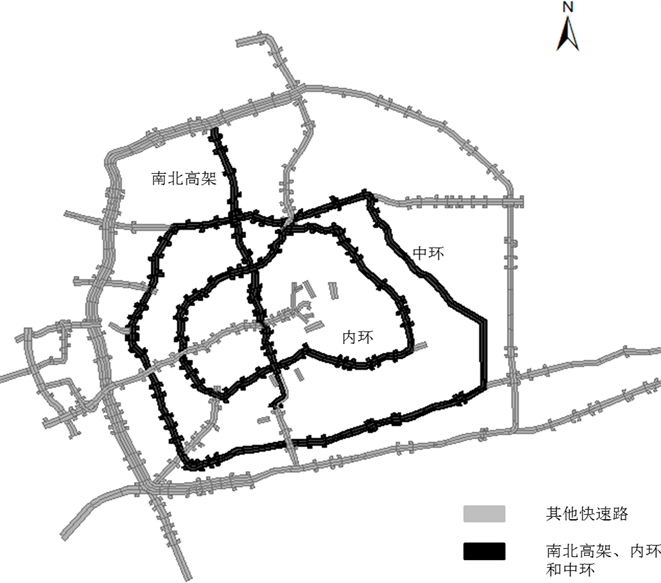Figure 2. Shanghai urban freeway networkTable 2. The time-varying condition of traffic congestion index of the road networkTable 3. Subjective and objective weight and influence importance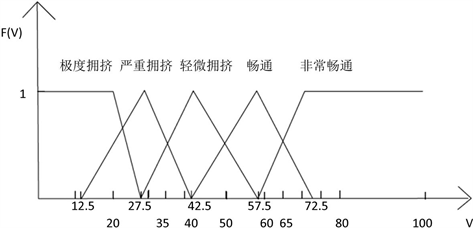Figure 3. Membership function of velocity

${u}_{5}\left(V\right)=\left\{\begin{array}{l}1,\text{\hspace{0.17em}}\text{\hspace{0.17em}}\text{\hspace{0.17em}}\text{\hspace{0.17em}}\text{\hspace{0.17em}}\text{\hspace{0.17em}}\text{\hspace{0.17em}}\text{\hspace{0.17em}}\text{\hspace{0.17em}}\text{\hspace{0.17em}}\text{\hspace{0.17em}}\text{\hspace{0.17em}}\text{\hspace{0.17em}}\text{\hspace{0.17em}}\text{\hspace{0.17em}}\text{\hspace{0.17em}}\text{\hspace{0.17em}}\text{\hspace{0.17em}}\text{\hspace{0.17em}}\text{\hspace{0.17em}}\text{\hspace{0.17em}}\text{\hspace{0.17em}}V<20\\ 1-\frac{V-20}{7.5},\text{\hspace{0.17em}}\text{\hspace{0.17em}}\text{\hspace{0.17em}}20${u}_{3}\left(V\right)=\left\{\begin{array}{l}0,\text{\hspace{0.17em}}\text{\hspace{0.17em}}\text{\hspace{0.17em}}\text{\hspace{0.17em}}\text{\hspace{0.17em}}\text{\hspace{0.17em}}\text{\hspace{0.17em}}\text{\hspace{0.17em}}\text{\hspace{0.17em}}\text{\hspace{0.17em}}\text{\hspace{0.17em}}\text{\hspace{0.17em}}\text{\hspace{0.17em}}\text{\hspace{0.17em}}\text{\hspace{0.17em}}\text{\hspace{0.17em}}\text{\hspace{0.17em}}\text{\hspace{0.17em}}\text{\hspace{0.17em}}\text{\hspace{0.17em}}\text{\hspace{0.17em}}\text{\hspace{0.17em}}\text{\hspace{0.17em}}\text{\hspace{0.17em}}\text{\hspace{0.17em}}V<27.5\\ \frac{V-27.5}{15},\text{\hspace{0.17em}}\text{\hspace{0.17em}}\text{\hspace{0.17em}}\text{\hspace{0.17em}}\text{\hspace{0.17em}}\text{\hspace{0.17em}}\text{\hspace{0.17em}}\text{\hspace{0.17em}}\text{\hspace{0.17em}}27.5

${u}_{2}\left(V\right)=\left\{\begin{array}{l}0,\text{\hspace{0.17em}}\text{\hspace{0.17em}}\text{\hspace{0.17em}}\text{\hspace{0.17em}}\text{\hspace{0.17em}}\text{\hspace{0.17em}}\text{\hspace{0.17em}}\text{\hspace{0.17em}}\text{\hspace{0.17em}}\text{\hspace{0.17em}}\text{\hspace{0.17em}}\text{\hspace{0.17em}}\text{\hspace{0.17em}}\text{\hspace{0.17em}}\text{\hspace{0.17em}}\text{\hspace{0.17em}}\text{\hspace{0.17em}}\text{\hspace{0.17em}}\text{\hspace{0.17em}}\text{\hspace{0.17em}}\text{\hspace{0.17em}}\text{\hspace{0.17em}}\text{\hspace{0.17em}}V<42.5\\ \frac{V-42.5}{15},\text{\hspace{0.17em}}\text{\hspace{0.17em}}\text{\hspace{0.17em}}\text{\hspace{0.17em}}\text{\hspace{0.17em}}\text{\hspace{0.17em}}\text{\hspace{0.17em}}42.5

${u}_{1}\left(V\right)=\left\{\begin{array}{l}0,\text{\hspace{0.17em}}\text{\hspace{0.17em}}\text{\hspace{0.17em}}\text{\hspace{0.17em}}\text{\hspace{0.17em}}\text{\hspace{0.17em}}\text{\hspace{0.17em}}\text{\hspace{0.17em}}\text{\hspace{0.17em}}\text{\hspace{0.17em}}\text{\hspace{0.17em}}\text{\hspace{0.17em}}\text{\hspace{0.17em}}\text{\hspace{0.17em}}\text{\hspace{0.17em}}\text{\hspace{0.17em}}\text{\hspace{0.17em}}\text{\hspace{0.17em}}\text{\hspace{0.17em}}V<57.5\\ \frac{V-57.5}{7.5},\text{\hspace{0.17em}}\text{\hspace{0.17em}}\text{\hspace{0.17em}}57.5

$\left[\begin{array}{ccc}V& SP& TP\end{array}\right]=\left[\begin{array}{ccc}56.4& 10.25%& 9.67%\end{array}\right]$

$X={\text{e}}^{R}=\left[\begin{array}{ccccc}1& 2.5191& 1.0791& 1& 1\\ 1.0249& 1.6691& 1& 1& 1\\ 2.7183& 1.6216& 1& 1& 1\end{array}\right]$Table 4. The evaluation results of congestion level under different methodsFigure 4. Road network average flow-average density diagram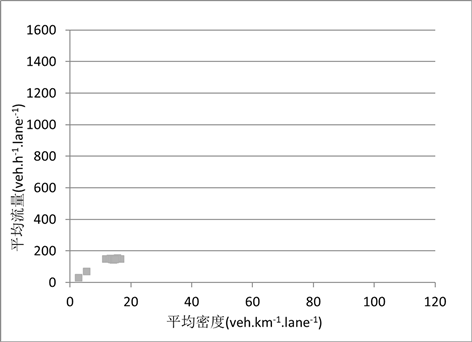Figure 5. Average flow-average density of the road network after the upper limit of the coordinate axis is extended to the standard range

5. 结论

An Improved Non-Linear Fuzzy Comprehensive Method for Assessing Urban Freeway Network Traffic Congestion Level[J]. 交通技术, 2020, 09(02): 68-78. https://doi.org/10.12677/OJTT.2020.92009

1. 1. 余叶. 上海: 快速路只占5%道路面积承担30%交通流量[EB/OL]. http://news.chengdu.cn/content/2012-02/07/content_922835.htm?node=487, 2012-02-07/2013-01-13.

2. 2. 北京日报. 解读北京快速路: 8％的道路承载50％的流量[EB/OL]. http://news.sohu.com/20060113/n241418163.shtml, 2006-01-13/2020-01-13.

3. 3. 李伟, 方怡沁, 杨鸣. 公路网运行拥堵状态定量化评价指标测算与发布的国内外经验借鉴[J]. 公路, 2019, 64(7): 218-224.

4. 4. 白骅, 王建军, 蒋意眉. 基于点、线、面层次的公路网交通运行状态评价[J]. 中国公路学报, 2018, 31(11): 197-204.

5. 5. 罗蓓蓓. 拥挤管理系统(CMS)实施方法研究[D]: [硕士学位论文]. 上海: 同济大学, 2008.

6. 6. 钟秀. 偶发性大范围拥堵与常态性拥堵特征差异分析[D]: [硕士学位论文]. 上海: 同济大学, 2010.

7. 7. 李晓丹, 刘好德, 杨晓光, 等. 城市道路网络交通状态时空演化量化分析[J]. 系统工程, 2008(12): 66-70.

8. 8. 任其亮. 时空路网交通拥堵预测与疏导决策方法研究[D]: [博士学位论文]. 成都: 西南交通大学, 2007.

9. 9. 郭继孚, 刘梦涵, 于雷, 等. 北京市交通拥堵宏观评价指标体系开发及其应用[C]//科学技术部全国智能运输系统协调指导小组办公室. 2007第三届中国智能交通年会论文集. 南京: 东南大学出版社, 2007: 8.

10. 10. 吉静, 顾承华, 翟希, 等. 基于交通状态指数的城市道路交通拥堵评价体系研究[C]//中国智能交通协会. 第十一届中国智能交通年会大会论文集. 北京: 电子工业出版社, 2016: 931-939.

11. 11. 龚峻峰, 苏奎, 何兆成, 等. 广州市路网交通状态评价方法及其在亚运交通管控分析中的应用[C]//中国智能交通协会. 第六届中国智能交通年会暨第七届国际节能与新能源汽车创新发展论坛优秀论文集(上册)——智能交通. 北京: 科学技术文献出版社, 2011: 2.

12. 12. Hamad, K. and Kikuchi, S. (2002) Developing a Measure of Traffic Congestion: Fuzzy Inference Approach. Transportation Research Record, 1802, 77-85.

13. 13. 戢晓峰. 基于交通信息提取的区域路网拥挤管理方法[D]: [博士学位论文]. 成都: 西南交通大学, 2009.

14. 14. 刘海青, 杨立才, 吴磊, 等. 基于Fuzzy-PCA的城市区域交通拥挤评价方法[J]. 山东大学学报(工学版), 2012(6): 56-62.

15. 15. 刘亚非. 基于多源数据的城市区域路网交通状态评价[D]: [硕士学位论文]. 上海: 同济大学, 2012.

16. 16. 胡庆和, 施国庆, 黄涛珍, 等. 非线性FCA模型在流域水资源冲突风险评价中的应用[J]. 水利水电科技进展, 2007(2): 6-9.

17. 17. 胡娟, 刘志斌. 油田开发规划的非线性模糊综合评价模型[J]. 石油天然气学报, 2011, 33(10): 132-135 + 169.

18. 18. 北京市市政工程设计研究总院. CJJ 129-2009城市快速路设计规程[S]. 北京: 中国建筑工业出版社, 2009.

19. 19. 张晓慧, 冯英浚. 一种非线性模糊综合评价模型[J]. 系统工程理论与实践, 2005, 25(10): 54-59.

20. 20. 朱琳, 于雷, 宋国华. 基于MFD的路网宏观交通状态及影响因素研究[J]. 华南理工大学学报(自然科学版), 2012, 40(11): 138-146.

21. 21. 姚崇富, 付强, 林航飞. 上海快速路网宏观基本图特征研究[J]. 武汉理工大学学报(交通科学与工程版), 2016, 40(3): 519-525.

22. 22. 丁恒, 朱良元, 蒋程镔, 等. 基于宏观基本图的快速路网交通状态识别方法[J]. 重庆交通大学学报(自然科学版), 2018, 37(12): 77-83.